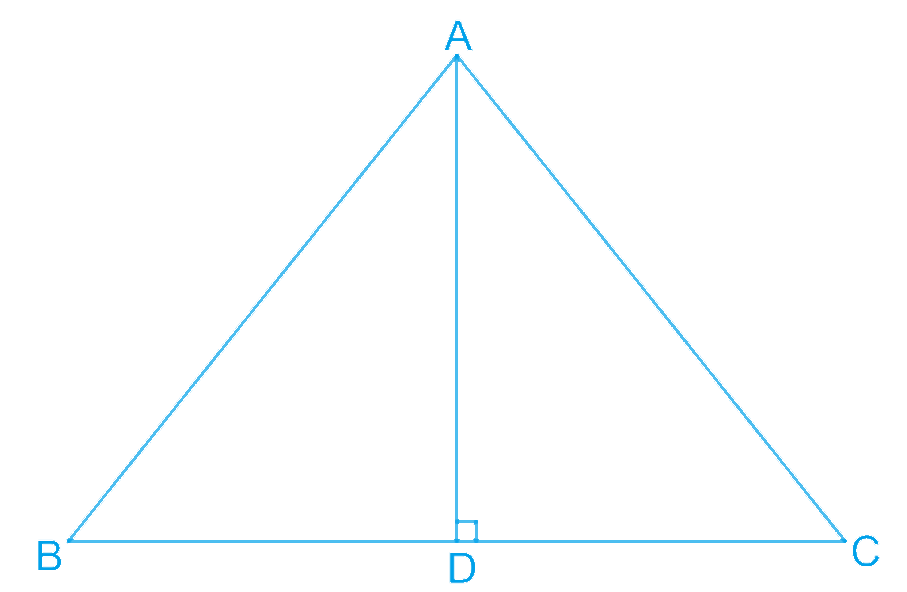# Ex.7.2 Q2 Triangles Solution - NCERT Maths Class 9

Go back to  'Ex.7.2'

## Question

In $$\Delta ABC, AD$$ is the perpendicular bisector of $$BC$$ (see the given figure). Show that $$\Delta ABC$$ is an isosceles triangle in which $$AB = AC$$.

Video Solution
Triangles
Ex 7.2 | Question 2

## Text Solution

What is Known?

$$AD$$ is perpendicular bisector of $$BC$$ means and $$BD=DC$$

To prove:

$$\Delta ABC$$ is an isosceles triangle in which $$AB = AC.$$

Reasoning:

We can show two triangles ADB and ADC congruent by using SAS congruency rule and then we can say corresponding parts of congruent triangles will be equal.Steps:

In $$\Delta ADC\text{ and }\Delta ADB,$$

\begin{align}&AD=AD (Common)\\&\angle ADC=\angle ADB\ (\text{Each }\!\!~\!\!\text{ }{{90}^{{}^\circ }})\\&CD=BD\\&\left( \begin{array} & \text{AD is the perpendicular } \\ \text{bisector of BC} \\ \end{array} \right)\\\\&\therefore \Delta ADC\cong \Delta ADB \\&\text{(By SAS congruence rule)}\\\\&\therefore AB=AC\,\,\,(\text{By }CPCT)\!\!~\!\!\end{align}

Therefore, $$ABC$$ is an isosceles triangle in which $$AB = AC$$.

Video Solution
Triangles
Ex 7.2 | Question 2

Learn from the best math teachers and top your exams

• Live one on one classroom and doubt clearing
• Practice worksheets in and after class for conceptual clarity
• Personalized curriculum to keep up with school